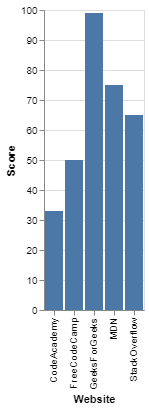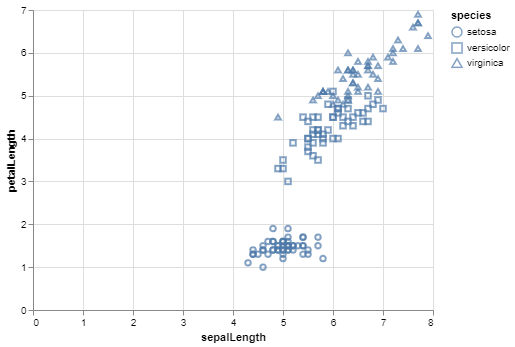Open In App

# Introduction to Altair in Python

Altair is a statistical visualization library in Python. It is a declarative in nature and is based on Vega and Vega-Lite visualization grammars. It is fast becoming the first choice of people looking for a quick and efficient way to visualize datasets. If you have used imperative visualization libraries like matplotlib, you will be able to rightly appreciate the capabilities of Altair.

It is rightly regarded as declarative visualization library since, while visualizing any dataset in Altair, the user only needs to specify how the data columns are mapped to the encoding channel i.e. declare links between the data columns and encoding channels such as x and y axis, row, columns, etc. Simply framing, a declarative visualization library allows you to focus on the “what” rather than the “how” part, by handling the other plot details itself without the users help.

On the contrary, Imperative libraries such as matplotlib force you to specify the “how” part of the visualization which takes away the focus from the data and the relationship between them. This also makes the code long and time-consuming as you have to specify details such as legends and axis names yourself.

### Installation

The following command can be used to install Altair like any other python library:

```pip install altair
```

We are going to use datasets from the vega_datasets package. To install, following command should be employed:

```pip install vega_datasets
```

Note- Jupyter Notebook should be used to execute the code as the visualizations require a Javascript frontend to display the charts. You can refer the following article to know how to use Jupyter Notebook: Getting Started with Jupyter Notebook. You can also use JupyterLab, Zeppelin or any other notebook environment or IDE with notebook support.

### Essential Elements of an Altair Chart

All altair charts need three essential elements: Data, Mark and Encoding. A valid chart can also be made by specifying only the data and mark.

The basic format of all altair chart is:

alt.Chart(data).mark_bar().encode(

encoding1 = ‘column1’,

encoding2 = ‘column2’,

)

• Make a chart.
• Pass in some data.
• Specify the type of mark you want.
• Specify the encoding.

Now, lets look at the essential elements in detail.

#### Data

The dataset is the first argument that you pass to the chart. Data in Altair is built around the Pandas Dataframe so the encoding becomes quite simple and it is able to detect the data types required in the encoding but you can also use the following for the data:

• A Data or related object such as UrlData, InlineData, NamedData
• A json or csv formatted text file or url
• An object that supports the __geo_interface__(eg. Geopandas GeoDataFrame, GeoJSON Objects)

Using DataFrames will make the process easier, so you should use DataFrames wherever possible.

#### Mark

Mark property specifies how the data should be represented on the plot. There are many types of mark methods available in Altair having the following format:

```mark_markname()
```

Some basic marks include area, bar, point, text, tick and line. Altair also provides some compound marks like box plot, error band and error bar. These mark methods can also accept optional arguments like color and opacity.

One of the main advantages of using Altair is that the chart type can be changed just by changing the mark type only.

#### Encoding

One of the most important things in visualization is the mapping of data to the visual properties of the chart. This mapping in Altair is called encoding and is carried out through the Chart.encode() method. There are various types of encoding channels available in Altair: position channels, mark property channels, hyperlink channels, etc. Out of these the most commonly used are the x(x-axis value) and y(y-axis value) from position channels and color and opacity from mark property channels.

1. The basic code remains the same for all types of plots, the user only needs to change the mark attribute to get different plots.
2. The code is shorter and simpler to write than other imperative visualization libraries. User can focus on the relationship between the data columns and forget about the unnecessary plot details.
3. Faceting and Interactivity are very easy to implement.

### Examples

Program 1 : (Simple Bar Chart)

## Python3

 `# Importing altair and pandas library``import` `altair as alt``import` `pandas as pd`` ` `# Making a Pandas DataFrame``score_data ``=` `pd.DataFrame({``    ``'Website'``: [``'StackOverflow'``, ``'FreeCodeCamp'``,``                ``'GeeksForGeeks'``, ``'MDN'``, ``'CodeAcademy'``],``    ``'Score'``: [``65``, ``50``, ``99``, ``75``, ``33``]``})`` ` `# Making the Simple Bar Chart``alt.Chart(score_data).mark_bar().encode(``    ``# Mapping the Website column to x-axis``    ``x``=``'Website'``,``    ``# Mapping the Score column to y-axis``    ``y``=``'Score'``)`

Output:Simple Bar Chart using Altair

Program 2 : (Scatter Plot)

In this example, we will visualize the iris dataset from the vega_datasets library in the form of a scatter plot. The mark method used for scatter plot in this example is mark_point(). For this bi-variate analysis, we map the sepalLength and petalLength columns to the x and y axes encoding. Further, to differentiate the points from each other, we map the shape encoding to the species column.

## Python3

 `# Importing altair``import` `altair as alt``# Import data object from vega_datasets``from` `vega_datasets ``import` `data`` ` `# Selecting the data``iris ``=` `data.iris()`` ` `# Making the Scatter Plot``alt.Chart(iris).mark_point().encode(``    ``# Map the sepalLength to x-axis``    ``x``=``'sepalLength'``,``    ``# Map the petalLength to y-axis``    ``y``=``'petalLength'``,``    ``# Map the species to shape``    ``shape``=``'species'``)`

Output:Scatter Plot using Altair